Tamilnadu State Board New Syllabus Samacheer Kalvi 8th Maths Guide Pdf Chapter 1 Numbers Ex 1.4 Textbook Questions and Answers, Notes.

## Tamilnadu Samacheer Kalvi 8th Maths Solutions Chapter 1 Numbers Ex 1.4

Question 1.
Fill in the blanks:
(i) The ones digit in the square of 77 is ________ .
9

(ii) The number of non-square numbers between 242 and 252 is ________ .
48(iii) The number of perfect square numbers between 300 and 500 is ________ .
5

(iv) If a number has 5 or 6 digits in it, then its square root will have ________ digits.
3

(v) The value of Jii lies between integers ______ and ________ .
13, 14

Question 2.
Say True or False:
(i) When a square number ends in 6, its square root will have 6 in the unit’s place.
True

(ii) A square number will not have odd number of zeros at the end.
True

(iii) The number of zeros in the square of 91000 is 9.
False

(iv) The square of 75 is 4925.
False

(v) The square root of 225 is 15.
TrueQuestion 3.
Find the square of the following numbers.
(i) 17
(ii) 203
(iii) 1098
(i) 17(ii) 203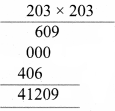(iii) 1098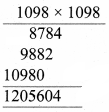Question 4.
Examine if each of the following is a perfect square.
(i) 725
(ii) 190
(iii) 841
(iv) 1089
(i) 725
725 = 5 × 5 × 29 = 52 × 29
Here the second prime factor 29 does not have a pair.
Hence 725 is not a perfect square number.(ii) 190
190 = 2 × 5 × 19
Here the factors 2, 5 and 9 does not have pairs.
Hence 190 is not a perfect square number.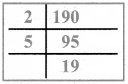(iii) 841
841 = 29 × 29
Hence 841 is a perfect square

(vi) 1089
1089 = 3 × 3 × 11 × 11 = 33 × 33
Hence 1089 is a perfect square

The factors of 144 are 1, 2, 3, 4, 6, 8, 9, 12, 16, 18, 24, 36, 48, 72, 144.

Question 5.
Find the square root by prime factorisation method.
(i) 144
(ii) 256
(iii) 784
(iv) 1156
(v) 4761
(vi) 9025
(i) 144
144 = 2 × 2 × 2 × 2 × 3 × 3
√144 = 2 × 2 × 3 = 12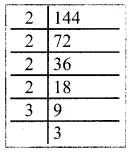(ii) 256
256 = 2 × 2 × 2 × 2 × 2 × 2 × 2 × 2
√256 = 2 × 2 × 2 × 2 = 16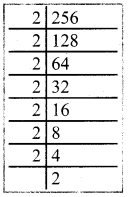(iii) 784
784 = 2 × 2 × 2 × 2 × 7 × 7
√784 = 2 × 2 × 2 × 2 × 7 × 7 = 28(iv) 1156
1156 = 2 × 2 × 17 × 17
1156 = 22 × 172
1156 = (2 × 17)2
∴ $$\sqrt{1156}$$ = $$\sqrt{(2 \times 17)^{2}}$$ = 2 × 17 = 34
∴ $$\sqrt{1156}$$ = 34(v) 4761
4761 = 3 × 3 × 23 × 23
4761 = 32 × 232
4761 = (3 × 23)2
√4761 = $$\sqrt{(3 \times 23)^{2}}$$
√4761 = 3 × 23
√4761 = 69(vi) 9025
9025 = 5 × 5 × 19 × 19
9025 = 52 × 192
9025 = (5 × 19)2
√925 = $$\sqrt{(5 \times 19)^{2}}$$ = 5 × 19 = 95Question 6.
Find the square root by long division method.
(i) 1764
(ii) 6889
(iii) 11025
(iv) 17956
(v) 418609
(i) 1764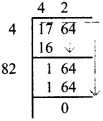√1764 = 42

(ii) 6889√6889 = 83(iii) 11025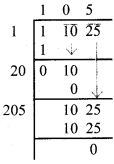√11025 = 105

(iv) 17956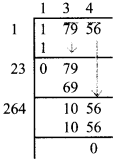√17956 = 134

(v) 418609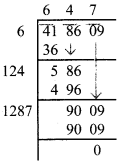√418609 = 647Roots Calculator is a free online tool that displays the roots of the given quadratic equation.

Question 7.
Estimate the value of the following square roots to the nearest whole number:
(i) √440
(ii) √800
(iii) √1020
(i) √440
we have 202 = 400
212= 441
∴ √440 ≃ 21

(ii) √800
we have 282 = 784
292= 841
∴ √800 ≃ 28

(iii) √1020
we have 312 = 961
322= 1024
∴ √1020 ≃ 32Question 8.
Find the square root of the following decimal numbers and fractions.
(i) 2.89
(ii) 67.24
(iii) 2.0164
(iv) $$\frac{144}{225}$$
(v) $$7 \frac{18}{49}$$
(i) 2.89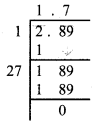√2.89 = 1.7

(ii) 67.24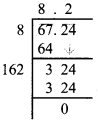√67.24 = 8.2

(iii) 2.0164√2.0164 = 1.42(iv) $$\frac{144}{225}$$(v) $$7 \frac{18}{49}$$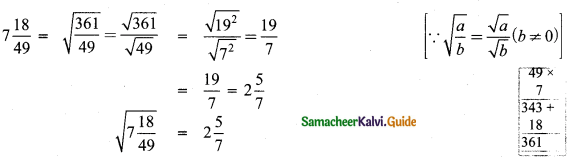$$\sqrt{7 \frac{18}{49}}=2 \frac{5}{7}$$

Question 9.
Find the least number that must be subtracted to 6666 so that it becomes a perfect square. Also, find the square root of the perfect square thus obtained.
Let us work out the process of finding the square root of 6666 by long division method.The remainder in the last step is 105. Is if 105 be subtracted from the given number the
remainder will be zero and the new number will be a perfect square.
∴ The required number is 105. The square number is 6666 – 105 = 6561.Question 10.
Find the least number by which 1800 should be multiplied so that it becomes a perfect square. Also, find the square root of the perfect square thus obtained.
We find 1800 = 2 × 2 × 3 × 3 × 5 × 5 × 2
= 22 × 32 × 52 × 2
Here the last factor 2 has no pair. So if we multiply 1800 by 2, then the number becomes a perfect square.∴ 1800 × 2 = 3600 is the required perfect square number.
∴ 3600 = 1800 × 2
3600 = 22 × 32 × 52 × 2 × 2
3600 = 22 × 32 × 52 × 22
= (2 × 3 × 5 × 2)2
$$\sqrt{3600}=\sqrt{(2 \times 3 \times 5 \times 2)^{2}}$$
= 2 × 3 × 5 × 2 = 60
∴ √3600 = 60Objective Type Questions

Question 11.
The square of 43 ends with the digit .
(A) 9
(B) 6
(C) 4
(D) 3
(A) 9
Hint:
Ones digit = 3 × 3 = 9

Question 12.
_______ is added to 242 to get 252.
(A) 42
(B) 52
(C) 62
(D) 72
(D) 72
Hint:
252 = 25 × 25 = 625
242 = 24 × 24 = 576Question 13.
√48 is approximately equal to .
(A) 5
(B) 6
(C) 7
(D) 8
(C) 7
Hint:
√49 = 7

Question 14.
$$\sqrt{128}-\sqrt{98}+\sqrt{18}$$
(A) √2
(B) √8
(C) √48
(D) √32
(D) √32Question 15.
The number of digits in the square root of 123454321 is ______.
(A) 4
(B) 5
(C) 6
(D) 7
$$\frac{n+1}{2}=\frac{10}{2}=5$$